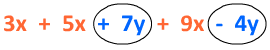# Combining Like Terms

In order to solve equations or simplify expressions, you may need to combine "like terms".

For example, say you have the expression 3x + 5x + 7y + 9x - 4y.

This expression looks a bit confusing, but we can combine common terms to make it much simpler.

To be a common term, the term must have the same variable and the same exponents.When you combine like terms, be sure to use the + or - that is in front of the coefficient, or number in before the letter.

So in this case, we will add the 3, 5 and 9 that is in front of the x terms. Then we will subtract 7 and 4 that is in front of the y terms.

3x + 5x + 7y + 9x - 4y = 17x + 3y

Here are a few more examples:

1.) 7m + 14m - 6n - 5n + 2m

Step 1: Organize your like terms. You can use a highlighter, shapes, or just rewrite the problem so that the like terms are next to each other.

7m + 14m - 6n - 5n + 2m

Step 2: Combine the coefficients.

(7 + 14 + 2)m + (-6 + -5)n

23m - 11n

2.) 5h + 11g + h - 8g

Step 1: Organize your like terms.

5h + 11g + h - 8g

Step 2: Combine the coefficients.

When you have a variable that does not have a coefficient, you may write

5h + 11g + 1h - 8g

(5 + 1)h + (11 - 8)g

6h + 3g

3.) 5x + 8x + 10x2 - 7x2 - 4x

Step 1: Organize your like terms.

This problem often leads to a common mistake. Just because they all
have x in them does not mean that they are all like terms.
The variable AND the exponent must be the same.

5x + 8x + 10x2 - 7x2 - 4x

Step 2: Combine the coefficients

(5 + 8 - 4)x + (10 - 7)x2

9x + 3x2